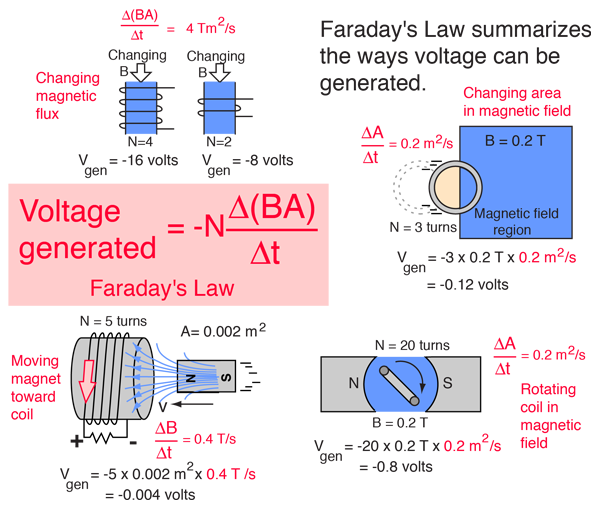The concept of Faraday's Law is that any change in the magnetic environment of a coil of wire will cause a voltage (emf) to be "induced" in the coil. No matter how the change is produced, the voltage will be generated. The change could be produced by changing the magnetic field strength, moving a magnet toward or away from the coil, moving the coil into or out of the magnetic field, rotating the coil relative to the magnet, etc.At upper left in the illustration, two coils are penetrated by a changing magnetic field. Magnetic flux F is defined by F=BA where B is the magnetic field or average magnetic field and A is the area perpendicular to the magnetic field. Note that for a given rate of change of the flux through the coil, the voltage generated is proportional to the number of turns N which the flux penetrates. This example is relevant to the operation of transformers, where the magnetic flux typically follows an iron core from the primary coil to the secondary coil and generates a secondary voltage proportional to the number of turns in the secondary coil.

Proceeding clockwise, the second example shows the voltage generated when a coil is moved into a magnetic field. This is sometimes called "motional emf", and is proportional to the speed with which the coil is moved into the magnetic field. That speed can be expressed in terms of the rate of change of the area which is in the magnetic field.

The next example is the standard AC generator geometry where a coil of wire is rotated in a magnetic field. The rotation changes the perpendicular area of the coil with respect to the magnetic field and generates a voltage proportional to the instantaneous rate of change of the magnetic flux. For a constant rotational speed, the voltage generated is sinusoidal.

The final example shows that voltage can be generated by moving a magnet toward or away from a coil of wire. With the area constant, the changing magnetic field causes a voltage to the generated. The direction or "sense" of the voltage generated is such that any resulting current produces a magnetic field opposing the change in magnetic field which created it. This is the meaning of the minus sign in Faraday's Law, and it is called Lenz's law.

Index